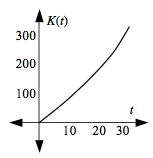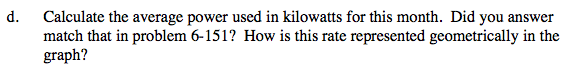### Home > CALC > Chapter 6 > Lesson 6.4.3 > Problem6-160

6-160.
1. The function k(t) = 8 − cos t + 0.01t2 + 0.01t helped Kira determine the rate at which electricity was being used on any day t of the month. However, to manage total electrical production, Kira needs to determine a function that will accumulate the energy used over the course of the month. That is, she needs a function K(t) that will determine the total energy used from the beginning of the month to time t. Homework Help ✎

1. Find a function K(t) that will accumulate the power used over time t. Be sure that K(0) = 0. What is its relationship to k(t)?

2. Use K(t) to find the total electrical energy used during this month.

3. Sketch a graph of K(t) for this 30-day period.

4. Calculate the average power used in kilowatts for this month. Did your answer match that in problem 6-151? How is this rate represented geometrically in the graph?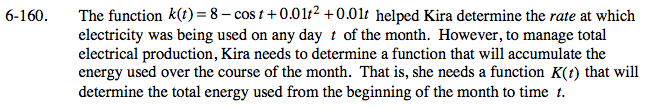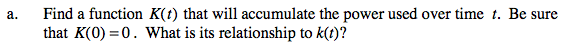Notice that lower-case, k(t), is a rate function.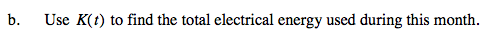Assume the month has 30 days.

'Total electrical consumption' is another way to say 'accumulated power.'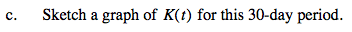Make sure that this graph agrees with your function for K(t).